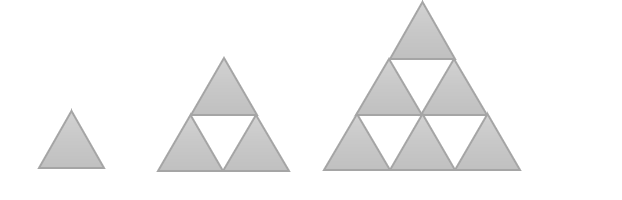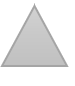Mathematics
Easy

Question

# Sophia made a pattern using triangles.If this pattern continues, the number oftriangles present in the next shape are____.

## 10    11    12    13Hint:

## The correct answer is: 11

### The number of triangles in first shape is 1, second shape is 3, third shape is 9.First shape has 1 triangle.Second shape has 3 triangle. It has two triangles more that first triangle.Third shape has 6 triangle. It has three more triangles than second.We are asked to find number of triangles in next shape.Fourth shape will have four more triangles.The number of triangles = 6 + 4= 10The number of triangles are 10

The main part of the question is to find the rule used to write the pattern.

### Related Questions to study#### With Turito Foundation.#### Get an Expert Advice From Turito.